## Android: Allowing users to pick 3D objects

I have published some apps on android but up until now I have never done anything with OpenGL, so thought it was about high time I get started. Bring on my first 2d game, I read a book and followed the examples.. and let me tell you that was fun. Then I decided I wanted to do my first 3d game, bring on cube destroy!! (not yet released or finished).

I was not far in to programming my first 3d game before I wanted users to be able to click on objects in 3d space, I was not able to find a complete tutorial on how to do this. After some time I used any resources I could and managed to get it working.. hence this blog to save you time!

So the basic process is broken up as follows:

1. Create a ray in 3d space based on a user click
2. Transform any of your 3d objects using the Model view matrix.
3. Check what objects are in the same space as the ray.
4. Find the closest object that was clicked if any.

The first thing you need is the coordinates of the click on the screen (screen ordinates and not 3d space ordinates). For those who are unaware the following on method on your view class will get these.

```
@Override
public boolean onTouchEvent(MotionEvent e) {
// MotionEvent reports input details from the touch screen
// and other input controls. In this case, you are only
// interested in events where the touch position changed.

float x = e.getX();
float y = e.getY();

switch (e.getAction()) {
case MotionEvent.ACTION_UP:
// do something with them...
break;
}
}

```

To use these new coordinates we need to convert them to space coordinates. Lucky for us there is a GLU method that will do this for us (http://developer.android.com/reference/android/opengl/GLU.html). Unlucky for us its not so easy to use, it requires the Model Matrix and View Matrix in order to do the conversions.

The hard part is getting hold of these Matrices, once again the developers at google have come to the party. If you open up the API demo's you will find a package com.example.android.apis.graphics.spritetext, open it and "borrow" the follow classes:
• MatrixGrabber
• MatrixStack
• MatrixTrackingGL
To be able to use these classes you are going to have to set a GL Wrapper on your view. So do the following in your Activity:

```    /** Called when the activity is first created. */
@Override
public void onCreate(Bundle savedInstanceState)
{
super.onCreate(savedInstanceState);
view = new MyView(this);
view.setGLWrapper(new GLSurfaceView.GLWrapper() {
public GL wrap(GL gl) {
return new MatrixTrackingGL(gl);
}
});
setContentView(view);
}

```

So now getting the Model and View Matricies respectivly is as easy as follows:

```     MatrixGrabber matrixGrabber = new MatrixGrabber();
matrixGrabber.getCurrentState(gl);
matrixGrabber.mModelView;
matrixGrabber.mProjection;

```
It will get the Matrix at the current state of OpenGL, so you need acquire them after doing your transformations.

Now we can almost use GLU.gluUnProject. The argument we do not have but will need is the viewport. You would have set your viewport in your Renderer:

```     public void onSurfaceChanged(GL10 gl, int width, int height) {
gl.glViewport(0, 0, width, height);
//if you set your viewport as above then the argument for GLU.gluUnProject would be.
int[] viewport = {0, 0, width, height};
//rest of method
```

So now we can obtain our ray, all we need is to find the far and near points on the Z axis. I just created a Ray class to hold the near and far vector which had a constructor to create the points.
```

public Ray(GL10 gl, int width, int height, float xTouch, float yTouch) {
MatrixGrabber matrixGrabber = new MatrixGrabber();
matrixGrabber.getCurrentState(gl);

int[] viewport = {0, 0, width, height};

float[] nearCoOrds = new float;
float[] farCoOrds = new float;
float[] temp = new float;
float[] temp2 = new float;
// get the near and far ords for the click

float winx = xTouch, winy =(float)viewport - yTouch;

//        Log.d(TAG, "modelView is =" + Arrays.toString(matrixGrabber.mModelView));
//        Log.d(TAG, "projection view is =" + Arrays.toString( matrixGrabber.mProjection ));

int result = GLU.gluUnProject(winx, winy, 1.0f, matrixGrabber.mModelView, 0, matrixGrabber.mProjection, 0, viewport, 0, temp, 0);

Matrix.multiplyMV(temp2, 0, matrixGrabber.mModelView, 0, temp, 0);
if(result == GL10.GL_TRUE){
nearCoOrds = temp2 / temp2;
nearCoOrds = temp2 / temp2;
nearCoOrds = temp2 / temp2;

}

result = GLU.gluUnProject(winx, winy, 0, matrixGrabber.mModelView, 0, matrixGrabber.mProjection, 0, viewport, 0, temp, 0);
Matrix.multiplyMV(temp2,0,matrixGrabber.mModelView, 0, temp, 0);
if(result == GL10.GL_TRUE){
farCoOrds = temp2 / temp2;
farCoOrds = temp2 / temp2;
farCoOrds = temp2 / temp2;
}
this.P0 = farCoOrds;
this.P1 = nearCoOrds;
}
```

Something to note here is that the result is homogeneous so x,z,y,w. Just divide the arguments by w to get the arguments.

Ok now we have our ray!! now could be different depending on what objects you are displaying. After doing any transformations to your object you will want to obtain the Model View matrix. Once you have this matrix you can take the vectors that make up your object and multiply them by the Model View matrix, this will transform them to where they are in space when rendered.

My game was made up of cubes, this is how I convert the coordinates:
```
float[] convertedSquare = new float[Cube.CUBE_CO_ORDS.length];
float[] resultVector = new float;
float[] inputVector = new float;

for(int i =0; i < Cube.CUBE_CO_ORDS.length;i = i+3){
inputVector = Cube.CUBE_CO_ORDS[i];
inputVector = Cube.CUBE_CO_ORDS[i+1];
inputVector = Cube.CUBE_CO_ORDS[i+2];
inputVector = 1;
Matrix.multiplyMV(resultVector, 0, matrixGrabber.mModelView, 0, inputVector,0);
convertedSquare[i] = resultVector/resultVector;
convertedSquare[i+1] = resultVector/resultVector;
convertedSquare[i+2] = resultVector/resultVector;

}
```
Now we have our converted square and our ray, we need to check if the exist anywhere in the same space. Its been a while since Linear math at uni so I looked it up on the net, I managed to find a C implementation. So I converted it to Java and hey presto we just call the method for the intersection. Here is a link to the page I got the implementation off: http://softsurfer.com/Archive/algorithm_0105/algorithm_0105.htm#Segment-Plan

Here is the code for intersections:

```
public class Triangle {
public float[] V0;
public float[] V1;
public float[] V2;

public Triangle(float[] V0, float[] V1, float[] V2){
this.V0 =V0;
this.V1 = V1;
this.V2 = V2;
}

private static final float SMALL_NUM =  0.00000001f; // anything that avoids division overflow

// intersectRayAndTriangle(): intersect a ray with a 3D triangle
//    Input:  a ray R, and a triangle T
//    Output: *I = intersection point (when it exists)
//    Return: -1 = triangle is degenerate (a segment or point)
//             0 = disjoint (no intersect)
//             1 = intersect in unique point I1
//             2 = are in the same plane
public static int intersectRayAndTriangle(Ray R, Triangle T, float[] I)
{
float[]    u, v, n;             // triangle vectors
float[]    dir, w0, w;          // ray vectors
float     r, a, b;             // params to calc ray-plane intersect

// get triangle edge vectors and plane normal
u =  Vector.minus(T.V1, T.V0);
v =  Vector.minus(T.V2, T.V0);
n =  Vector.crossProduct(u, v);             // cross product

if (Arrays.equals(n, new float[]{0.0f,0.0f,0.0f})){           // triangle is degenerate
return -1;                 // do not deal with this case
}
dir =  Vector.minus(R.P1, R.P0);             // ray direction vector
w0 = Vector.minus( R.P0 , T.V0);
a = - Vector.dot(n,w0);
b =  Vector.dot(n,dir);
if (Math.abs(b) < SMALL_NUM) {     // ray is parallel to triangle plane
if (a == 0){                // ray lies in triangle plane
return 2;
}else{
return 0;             // ray disjoint from plane
}
}

// get intersect point of ray with triangle plane
r = a / b;
if (r < 0.0f){                   // ray goes away from triangle
return 0;                  // => no intersect
}
// for a segment, also test if (r > 1.0) => no intersect

float[] tempI =  Vector.addition(R.P0,  Vector.scalarProduct(r, dir));           // intersect point of ray and plane
I = tempI;
I = tempI;
I = tempI;

// is I inside T?
float    uu, uv, vv, wu, wv, D;
uu =  Vector.dot(u,u);
uv =  Vector.dot(u,v);
vv =  Vector.dot(v,v);
w =  Vector.minus(I, T.V0);
wu =  Vector.dot(w,u);
wv = Vector.dot(w,v);
D = (uv * uv) - (uu * vv);

// get and test parametric coords
float s, t;
s = ((uv * wv) - (vv * wu)) / D;
if (s < 0.0f || s > 1.0f)        // I is outside T
return 0;
t = (uv * wu - uu * wv) / D;
if (t < 0.0f || (s + t) > 1.0f)  // I is outside T
return 0;

return 1;                      // I is in T
}

}
```

Here is a utility class for Vectors:
```
public class Vector {
// dot product (3D) which allows vector operations in arguments
public static float dot(float[] u,float[] v) {
return ((u[X] * v[X]) + (u[Y] * v[Y]) + (u[Z] * v[Z]));
}
public static float[] minus(float[] u, float[] v){
return new float[]{u[X]-v[X],u[Y]-v[Y],u[Z]-v[Z]};
}
public static float[] addition(float[] u, float[] v){
return new float[]{u[X]+v[X],u[Y]+v[Y],u[Z]+v[Z]};
}
//scalar product
public static float[] scalarProduct(float r, float[] u){
return new float[]{u[X]*r,u[Y]*r,u[Z]*r};
}
// (cross product)
public static float[] crossProduct(float[] u, float[] v){
return new float[]{(u[Y]*v[Z]) - (u[Z]*v[Y]),(u[Z]*v[X]) - (u[X]*v[Z]),(u[X]*v[Y]) - (u[Y]*v[X])};
}
//mangnatude or length
public static float length(float[] u){
return (float) Math.abs(Math.sqrt((u[X] *u[X]) + (u[Y] *u[Y]) + (u[Z] *u[Z])));
}

public static final int X = 0;
public static final int Y = 1;
public static final int Z = 2;
}
```

Ok so now all we need to do is call the method above and store the intersection, the shortest intersection vector will be the object they clicked!

sorry this is a bit rushed, I will come back and brush it up soon. Hope it helps someone?

1.Hi, I follow the steps, but unfortunately it does not work for me !!! may you please show an example of how to use triangle intersection method?

1.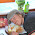what is not working for you?

2.This comment has been removed by the author.

3.And now it works !!!! thank you so much !!!

i have made some mistakes, i fixed them and now it works

2.i am trying to implement this ray picking on android sample on TouchRotate. but i am still don't understand on ray class and intersection. where should i place this class

thank and sorry for my bad english

3.Hi. Are you still active here?

4.yip I am active

5.Im trying to convert your code to PC OpenGL.
I almost made, and i hope it works, but i don't know until i draw cubes with correct coordinates.

Im getting near and far ray values but im not sure if they are correct.
How much experience you have with "PC" OpenGL?

And can you please show your Cube.CUBE_CO_ORDS array or whatever you have there and how you fill it. I can then reproduce this in my app and see , if my triangle intersection function will return TRUE finally.

Please. There is no example on the net how to convert ray in PC opengl to correct obj coords and your android example is only thing i can rely on.

TIA

6.And "convertedSquare[i], ..i+1, ..i+2" you are sending them to "intersectRayAndTriangle" as triangle vertex co-ordinates?

I dont know nothing about Android programming, but is your source in Java?

Like i said, it would be very helpful to see more pieces of your picking code. Or even better small OpenGL example app with your picking code.

7.I have no experience in pc opengl, all this code in a java.

8.This comment has been removed by a blog administrator.

9.This comment has been removed by the author.

10.Hi Gregory,

Nice work there. Thanks for sharing.

I am little bit stuck with the part on what to do once I have a Ray (P0, P1). My objects are spheres so I need to test if the Ray cut the sphere. Like how do I get the converted square and do I still use Triangle to test intersection. Any help would be appreciated.

Thanks

1.Nevermind. Figured out.

I created a test project to test your code on a cube. Worked well but sometimes I notice intersectRayAndTriangle returning 0 even when I click right on top of the cube. It might be something to do with the ModelViewMatrix. In my test project, I had onDrawFrame of Renderer class record current matrix state using matrixGrabber.getCurrentState(gl) as the last line.

2.Hi,

I am trying to use the above code to find which face of the cube I clicked. I am not understanding how to call intersectRayAndTriangle method. can you please help me to understand this or can you share your test project to test the code on a cube with me. My mail id is sushil.blore@gmail.com

11.Cab you please provide working sample code for it?

12.Sorry about the no replys... I have been away on holiday. I cannot place the whole example as its a game I made.

13.Thank you for detailed instructions, I've implemented picking objects by your tutorial.

However, I have a major recommendation for you. For your code to be applicable I've slightly modified it for better memory usage. It is very important in game not to allocate memory too much because GC causes lockups of process, and current implementations allocates a lot of memory.
For example, allocating new float[] on vector +/- operations is crazy.
I've modified it to return value into already created arrays, like this:

public class Vector
{
// dot product (3D) which allows vector operations in arguments
public static float dot(float[] u, float[] v) {
return ((u[X] * v[X]) + (u[Y] * v[Y]) + (u[Z] * v[Z]));
}

public static void minus(float[] u, float[] v, float[] result) {
result[X] = u[X] - v[X];
result[Y] = u[Y] - v[Y];
result[Z] = u[Z] - v[Z];
}

public static void addition(float[] u, float[] v, float[] result) {
result[X] = u[X] + v[X];
result[Y] = u[Y] + v[Y];
result[Z] = u[Z] + v[Z];
}

public static void scalarProduct(float r, float[] u, float[] result) {
result[X] = u[X] * r;
result[Y] = u[Y] * r;
result[Z] = u[Z] * r;
}

public static void crossProduct(float[] u, float[] v, float[] result) {
result[X] = (u[Y] * v[Z]) - (u[Z] * v[Y]);
result[Y] = (u[Z] * v[X]) - (u[X] * v[Z]);
result[Z] = (u[X] * v[Y]) - (u[Y] * v[X]);
}

// mangnatude or length
public static float length(float[] u) {
return (float) Math.abs(Math.sqrt((u[X] * u[X]) + (u[Y] * u[Y]) + (u[Z] * u[Z])));
}

public static final int X = 0;
public static final int Y = 1;
public static final int Z = 2;
}

Also there are quite a lot of allocations in static intersectRayAndTriangle() method, I've made a non-static version of it which uses pre-allocated float[] arrays.

1.I have not really had time to get back to my code and finish it off, will try in the coming year

14.Calculation of the intersections in 3d for a number of faces is very aggravating especially in Java. I've simplified the calculation of the coordinates of bringing in one plane and see if there is a touch point in the contour of the object. Then you can use to test the simple conditions ifelse

15.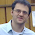I have copied the code and it really works. However, sometimes the code crashes. I get presenting "stack_underflow" exception when "MatrixTrackingGL.glPopMatrix"
is executed.

Do you have any idea how can I fix it ?

java.lang.IllegalArgumentException: stack underflow
at min3d.core.MatrixStack.glPopMatrix(MatrixStack.java:115)
at min3d.core.MatrixTrackingGL.glPopMatrix(MatrixTrackingGL.java:525)
at min3d.core.Renderer.drawObject(Renderer.java:527)
at min3d.core.Renderer.drawScene(Renderer.java:344)
at min3d.core.Renderer.onDrawFrame(Renderer.java:151)

1.I am at work so do not have my code in front of me, and can not remember if this is the error you get if you "pop" more times than you have "pushed"? maybe not.

16.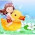Can you please tell me how can I use intersectRayAndTriangle() method with convertedSquare. By converting cube to Square what do you mean? How you are converting I do not understand? Please explain. After conversion I believe, the output are 6 squares, so how you are going to use it with triangle. I am new to OpenGL. I know cube can be created with Triangles. So do you mean to call intersectRayAndTriangle() method 2 times for the 2 triangles of each face of cube.
Any help will appreciated.

17.http://nehe.gamedev.net/article/using_gluunproject/16013/
In the above link they are not using "ray", but using
glReadPixels( x, int(winY), 1, 1, GL_DEPTH_COMPONENT, GL_FLOAT, &winZ );

Will it not work with Android? I am trying to use it. Please suggest.

18.Thanks a lot!, I think this is just what i need to get out of the hole i'm in.

But, there are some things i do not understand.

When you convert your cube, what's is going on there, do you get a normal vector of the cube like if it was a triangle? and what happens to those vectors? Are those the ones creating the triangle?.

Sorry for my english :P

Thanks a lot by the way for sharing.

19.Has anyone got a working sample of this? Sorry to ask but I am struggling to implement it. I have used colour picking before but would like to try this method
thanks
Simon

20.I actually have, i have correct math and info how to make ray picking and how to make it work for any nr of objects at any rotation or any coordinates.

I just have to port it to Java.

21.Hi Thanks for the tutorial! I am finding it a bit hard to find out where to actually put the code that you explained above. Can you tell me in class I should actually put the code blocks that you have shown here? or if you have a working example can you share it please??

1.Sorry there is a typo there.
*in which class I should actually put the code blocks?

22.hey, i have trouble with testing 3D ray picking...
Can i get some example source code by e-mail?
Here is my e-mail address : id_identity@naver.com

23.Fantatically useful post, thank you so much.

One issue I did find is that the view matrix has been applied twice to each ray endpoint. The multiplyMV transform shoild be removed, as the unproject already does this

Thanks again

1.Hy Kev , can you send to me application when you press the touchscreen, select a 3D object? min3d I set to work, and I loaded the 3d object obj mtl sites and texture, and I want to select an object when pressed tin touch an object, give me a menu arete for example ... can you help me?

24.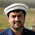25.Hi I'm just working my way through your tutorial and I'm just wondering where does the code for converting the co-ordinates go?

26.How do I implement this using GLES20? Will it work?

27.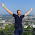+ Need implementation for GLES20, thanks!